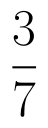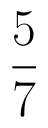Select Page

# CBSE Maths 12 Science Determinant MCQ Answers in English

CBSE Maths 12 Science Determinant MCQ Answers in English to enable students to get Answers in a narrative video format for the specific question.

Expert Teacher provides CBSE Maths 12 Science Determinant MCQ Answers through Video Answers in English language. This video solution will be useful for students to understand how to write an answer in exam in order to score more marks. This teacher uses a narrative style for a question from Determinant not only to explain the proper method of answering question, but deriving right answer too.

Please find the question below and view the Answer in a narrative video format.

Question:

## Similar Questions from CBSE, 12th Science, Maths, Determinant

Question 1 : Let A be a non-singular square matrix of order 3 * 3. Then | adj A | is equal to: (View Answer Video)

Question 2 : Evaluate. (View Answer Video)

Question 3 :  Find the values of x, if. (View Answer Video)

Question 4 : If, then x is equal to, (View Answer Video)

Question 5 : The following system of equations has x + 3y + 3z = 2, x + 4y + 3z = 1, x + 3y + 4z = 2,

### Probability

Question 1 : If the mean and variance of a Binomial distribution are 9 and 6 respectively, find the number of trails. (View Answer Video)

Question 2 : The probabilities of two students A and B coming to the school in time areandrespectively. Assuming that the events ' A coming in time ' and
' B coming in time ' are independent. Find the probability of only one. Write of them come in time atleast one advantage of coming to school in time.   (View Answer Video)

Question 3 : There are three coins. One is a two-headed coin (having head on both faces), another is a biased coin that comes up heads 75% of the times and third is also a biased coin that comes up tails 40% of the times. One of the three coins is chosen at random and tossed, and it shows heads. What is the probability that it was the two-headed coin?  (View Answer Video)

Question 4 : A bag contain 2 red, 6 black and 8 green balls. A ball is drawn at random from the bag. Find the probability of black balls:

Question 5 :  A card from a pack of 52 playing card is lost. From the remaining cards of the pack, three cards are drawn at random (without replacement) and are found to be all spades. Find the probability of the lost card being a spade.   (View Answer Video)

### Three Dimensional Geometry

Question 1 : Write the direction ratio's of the vectorwhereand(View Answer Video)

Question 2 : Find the angle between lineand the plane 2x - y + 2z -13 = 0. (View Answer Video)

Question 3 : Find the distance of the plane : 3x - 4y + 12z = 3 from the origin. (View Answer Video)

Question 4 : If a line makes angleswith the positive direction of co-ordinate axes, then write the value of(View Answer Video)

Question 5 : Find the distance between the point (7, 2, 4) and the plane determined by the points A(2, 5, -3), B(-2, -3, 5) and C(5, 3, -3). (View Answer Video)

### Application of Derivatives

Question 1 : Find two numbers whose sum is 24 and whose product is as large as possible. (View Answer Video)

Question 2 : A cylindrical tank of radius 10 m is being filled with wheat at the rate of 314 cubic meters per hour. Then the depth of the wheat is increasing at the rate of: (View Answer Video)

Question 3 : Equation of normal to the curve x+y=x^y where it cuts x-axis; is (View Answer Video)

Question 4 : Find two positive numbers x  and y such that x+y=60 and ismaximum. (View Answer Video)

Question 5 : The lineis a tangent to the curveat the point. (View Answer Video)